# Understanding Steering Behaviors: Flee and ArrivalThis post is part of a series called Understanding Steering Behaviors.
Understanding Steering Behaviors: Seek
Understanding Steering Behaviors: Wander

Steering behaviors aim to help autonomous characters move in a realistic manner, by using simple forces that combine to produce life-like, improvisational navigation. In this tutorial, I'll cover the flee behavior, which makes the character move away from a pursuer, and the arrival behavior, which makes the character slow down and stop as it approaches a target.

Note: Although this tutorial is written using AS3 and Flash, you should be able to use the same techniques and concepts in almost any game development environment. You must have a basic understanding of math vectors.

## Running Away

The seek behavior described previously is based on two forces that push the character towards the target: the desired velocity and the steering.

 1 desired_velocity = normalize(target - position) * max_velocity  2 steering = desired_velocity - velocity 

The desired_velocity, in this case, is the shortest path between the character and the target. It is calculated by subtracting the target's position from the character's position. The result is a force vector that goes from the character towards the target.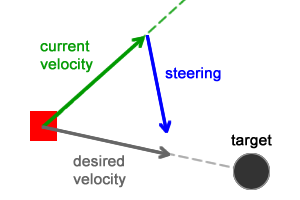Seek behavior

The flee behavior uses those same two forces, but they are adjusted to make the character run away from the target: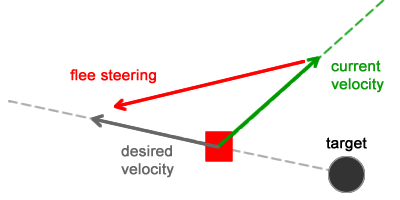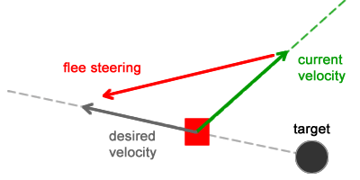Flee behavior

That new desired_velocity vector is calculated by subtracting the character's position from the target's position, which produces a vector that goes from the target towards the character

The resulting forces are calculated almost the same way as in the seek behavior:

 1 desired_velocity = normalize(position - target) * max_velocity  2 steering = desired_velocity - velocity 

The desired_velocity in that case represents the easiest escaping route the character can use to run away from the target. The steering force makes the character abandon its current route, pushing it towards the direction of the desired velocity vector.

Comparing the desired velocity vector of the flee behavior with the desired velocity vector of the seek behavior, the following relation can be established:

 1 flee_desired_velocity = -seek_desired_velocity 

In other words, one vector is the negative of the other.

After the steering force is calculated it must be added to the character's velocity vector. Since that force is pushing the character away from the target, every frame the character will stop moving towards the target and start moving away from it, producing a flee path (the orange curve in the figure below):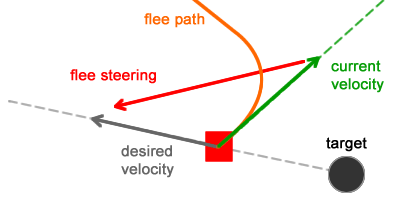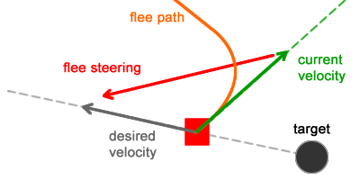The addition of those forces and the final velocity/position calculation are handled in the same way as before. Below is a demo showing several characters performing the flee behavior:

Move the mouse to move the target.

Every character is placed at the center of the moving area with a random velocity. They will try to flee from the target (the mouse cursor).  The addition of all forces makes each character smoothly abandon its current route and flee the target.

Currently the target affects every character, ignoring the distance from them; it could have been limited to an "effect area", where the character would flee only if it is close enough to the target.

## Arrival

The seek behavior, as we've seen, makes a character move towards a target. When it reaches the destination the steering force keeps acting on it based on the same rules, making the character "bounce" back and forth around the target.

The seek behavior. Move the mouse to move the target.

The arrival behavior prevents the character from moving through the target. It makes the character slow down as it approaches the destination, eventually stopping at the target.

The behavior is composed of two phases. The first phase is when the character is far away from the target and it works exactly the same way as the seek behavior does (move at full speed towards the target).

The second phase is when the character is close to the target, inside the "slowing area" (a circle centered at the target's position):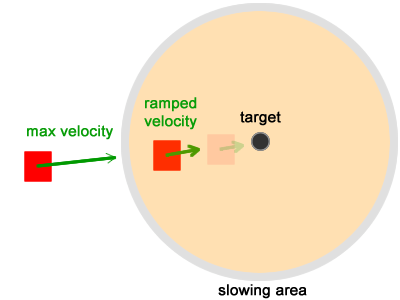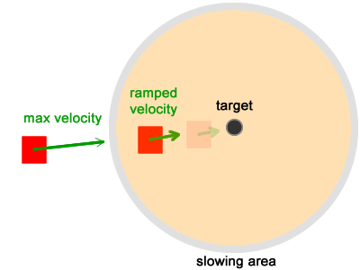When the character enters the circle, it slows down until it stops at the target.

## Slowing Down

When the character enters the slowing area its velocity is ramped down linearly to zero. This is achieved by adding a new steering force (the arrival force) to the character's velocity vector. The result of this addition will eventually become zero, meaning that nothing will be added to the character's position every frame (so there will be no movement):

 1 // If (velocity + steering) equals zero, then there is no movement  2 velocity = truncate(velocity + steering, max_speed)  3 position = position + velocity  4 5 function truncate(vector:Vector3D, max:Number) :void {  6  var i :Number;  7  i = max / vector.length;  8  i = i < 1.0 ? i : 1.0;  9  vector.scaleBy(i);  10 } 

In order to ensure the character will gradually slow down before it stops, the velocity should not become zero immediately. The gradual slow down process is calculated based on the radius of the slowing area and the distance between the character and the target:

 1 // Calculate the desired velocity  2 desired_velocity = target - position  3 distance = length(desired_velocity)  4 5 // Check the distance to detect whether the character  6 // is inside the slowing area  7 if (distance < slowingRadius) {  8  // Inside the slowing area  9  desired_velocity = normalize(desired_velocity) * max_velocity * (distance / slowingRadius)  10 } else {  11  // Outside the slowing area.  12  desired_velocity = normalize(desired_velocity) * max_velocity  13 }  14 15 // Set the steering based on this  16 steering = desired_velocity - velocity 

If the distance is greater than slowingRadius, it means the character is far away from the target and its velocity should remain max_velocity.

If the distance is less than slowingRadius, it means the character has entered the slowing area and its velocity should be ramped down.

The term distance / slowingRadius will vary from 1 (when distance equals slowingRadius) to 0 (when distance is almost zero). This linear variation will make the velocity smoothly slow down: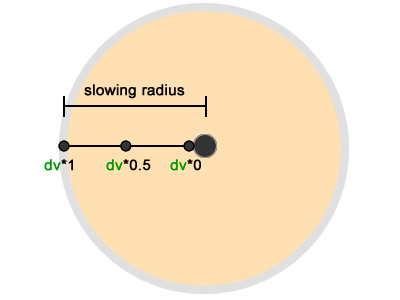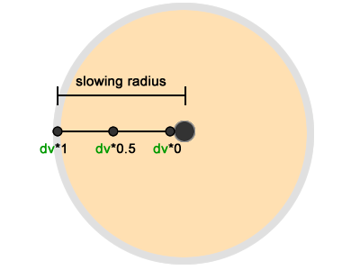As previously described, the character movement is performed as follows:

 1 steering = desired_velocity - velocity  2 velocity = truncate (velocity + steering , max_speed)  3 position = position + velocity 

If the desired velocity is ramped down to zero, then the steering force becomes -velocity. As a consequence, when that steering force is added to the velocity, it will result in zero, making the character stop moving.

Below is a demo showing the arrival behavior:

Move the mouse to move the target.

What the arrival behavior does, really, is calculate a force that will be equal to -velocity, preventing the character from moving as long as that force is in place. The character's original velocity vector does not change and it continues to work, but it is nulled by the steering addition.

If the arrival steering force is lifted, the character will start moving again, using its original velocity vector.

## Conclusion

The flee behavior makes the character move away from the desired target while the arrival behavior makes it slow down and stop at the target's position. Both can be used to create smooth escape or follow movement patterns, for instance. They can also be combined to create even more complex movements.

This tutorial described more about steering behaviors, explaining the flee and the arrival behaviors. Over the next few posts, we will learn about more behaviors. Keep up to date by following us on Twitter, Facebook, or Google+.